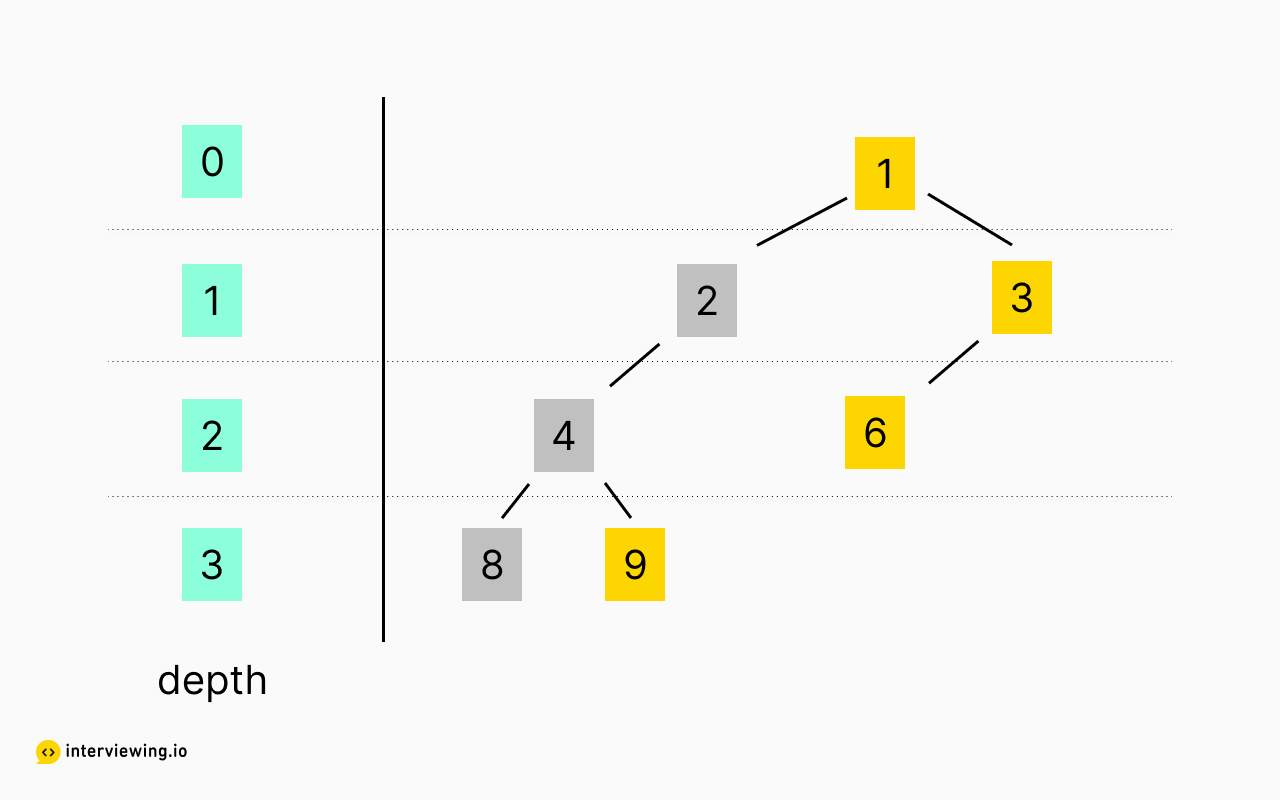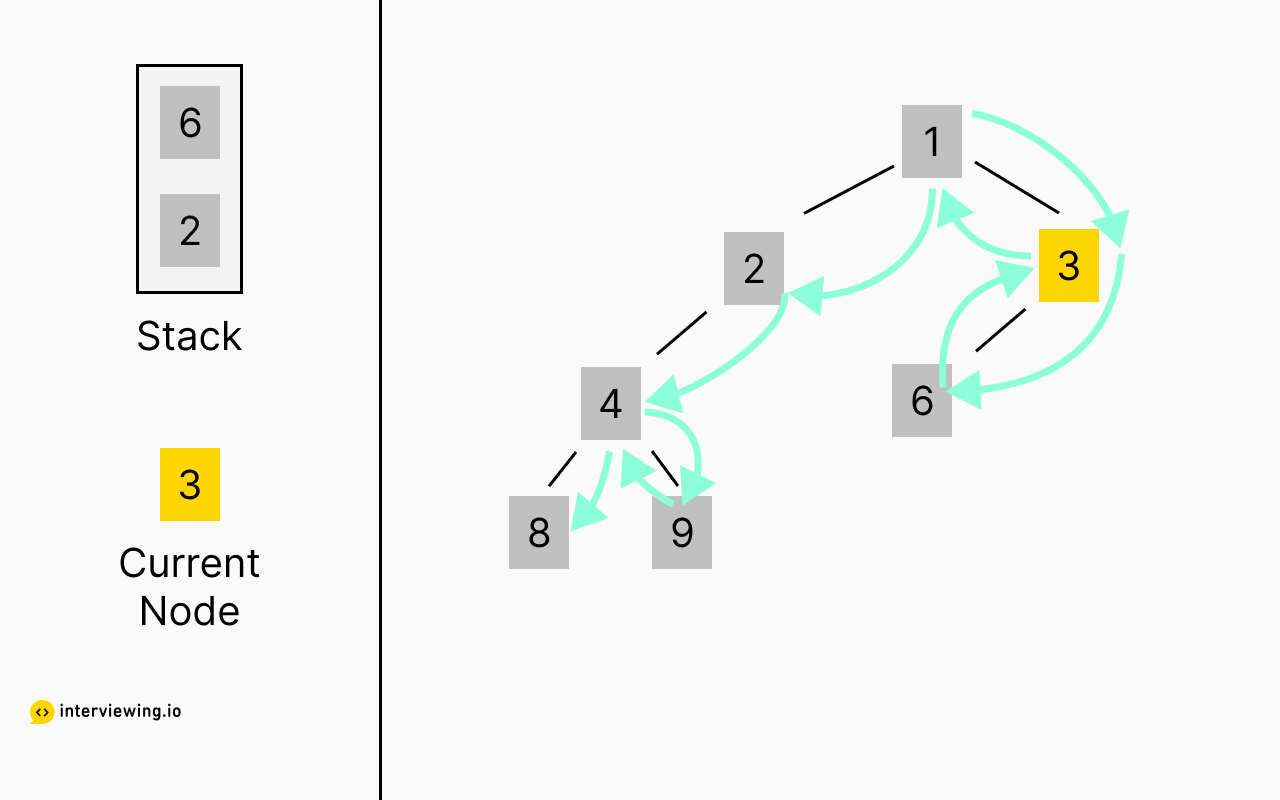MEDIUM
DATA STRUCTURES AND ALGORITHMS

# Interview Guide: How to Solve the Right View of a Binary Tree Problem

Written ByTom WagnerJared Skinner

## What is the Right Side View Of a Binary Tree Problem?

The Right View of Binary Tree problem involves taking a binary tree as input and returning a list of the nodes that would be visible to an observer positioned directly to the right of the tree. The problem demands a firm grasp of tree traversal algorithms in order to arrive at a linear time complexity solution.

## 3 Examples of the Right Side View Of a Binary Tree Problem

Given the root of a binary tree, imagine yourself standing on the right side of it. Return the values of the nodes you can see ordered from top to bottom.

Constraints

• The number of nodes in the tree is in the range `[0, 100]`
• `-100 <= Node.val <= 100`

### Example 1

input:

``````       0
/ \
1     2
/ \   / \
3   4 5   6``````

output: `[0, 2, 6]`

### Example 2

input:

``````       0
/
1
/ \
3   4``````

output: `[0, 1, 4]`

### Example 3

input:

``````       0
/ \
1     2
/     /
3     5``````

output: `[0, 2, 5]`

## How to Print the Right Side View Of a Binary Tree: with Solutions in Python, Java & JavaScript

We will present two different approaches to this problem. In the first approach, we will explore the nodes at each depth (distance from the root) and select the rightmost node. This type of algorithm is known as a breadth-first traversal. In the second approach, we will explore the tree from the right side to the left, drilling down as far as we can in each branch. This is known as a depth-first traversal.

### Approach 1: Layers Approach

For this first approach we will collect all nodes at each depth (we will refer to a collection of nodes at a given depth as a layer) . Once all nodes have been organized into layers we will select the rightmost node from each layer.

We will use a helper function that takes in a group of nodes and returns all child nodes. This will make collecting successive layers very easy!#### Right View of a Binary Tree Python, JavaScript and Java Solutions - Layers Approach

``````# Definition for a binary tree node.
# class TreeNode:
#     def __init__(self, val=0, left=None, right=None):
#         self.val = val
#         self.left = left
#         self.right = right

def get_children(nodes: list[Optional[TreeNode]]) -> list[Optional[TreeNode]]:
"""
Given a list of nodes, find all children nodes
"""
next_layer = []
for node in nodes:
if node.left != None:
next_layer.append(node.left)
if node.right != None:
next_layer.append(node.right)

return next_layer

def right_side_view(root: Optional[TreeNode]) -> List[int]:
if root == None:
return []

node_list = []

layers = []
next_layer = [root]
while next_layer != []:
layers.append(next_layer)
next_layer = get_children(next_layer)

for layer in layers:
node_list.append(layer[-1].val)

return node_list``````

#### Time and Space Complexity

• Time complexity: `O(n)` where `n` is the number of nodes.
• Space complexity: `O(n)` where `n` is the number of nodes.

Note: You might calculate the time/space complexity as `O(n+d)` where `d` is the depth of the tree since we need the additional `d` time/space for our algorithm. However, this complexity boils down to `O(n)` since `d <= n` which implies `O(n+d) <= O(2n) = O(n)`.

### Approach 2: Search Approach

Our space complexity is a little bloated since we are storing an additional copy of our tree when building the layers. Additionally, while `d` doesn't count against us in the complexities above, we can improve the real runtime of our solution. We are going to employ one of the most common tree-searching algorithms: Depth First Search (DFS). This algorithm works by drilling down a single branch of the tree until it reaches the bottom and then it backtracks until it finds a new branch. Proceeding this way we can inspect each node exactly once.

The algorithm works as follows:

• Create a stack `X` where nodes to explore will be kept.
• The root node is placed in `X`
• While `X` has something in it, pop a node `N` off `X`, find all children of `N`, and add them to `X`.

Note: Another implementation of this algorithm is to use recursion in place of a stack.

Note: This algorithm can be used for more than just binary trees, but if you employ this strategy in a graph with loops you also have to keep track of which nodes you have visited so you don't end up looking at the same nodes repeatedly!Now, we will employ this DFS algorithm and take the rightmost path first. While traversing we will also track how deep each node is. When inspecting a node, if we do not already have a node in our solution array at this depth, we will add this node. In this way, we will capture the right-most nodes of the tree.

#### Right View of a Binary Tree Python, JavaScript and Java Solutions - Search Approach

``````# Definition for a binary tree node.
# class TreeNode:
#     def __init__(self, val=0, left=None, right=None):
#         self.val = val
#         self.left = left
#         self.right = right

def right_side_view(root: Optional[TreeNode]) -> List[int]:
if root == None:
return []

node_list = []
stack = [(root, 0)]
while stack != []:
node, depth = stack.pop()
if node.left != None:
stack.append((node.left, depth+1))
if node.right != None:
stack.append((node.right, depth+1))

if depth >= len(node_list):
node_list.append(node.val)

return node_list``````

#### Time and Space Complexity

• Time complexity: `O(n)` where `n` is the number of nodes. We can't reduce this further since we need to check every node to know how deep the tree is.
• Space complexity: `O(d)` where `d` is the depth of the tree.

## Practice the Right View Of Binary Tree Problem With Our AI Interviewer

Start AI Interview